### Rose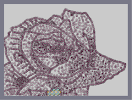Hover over the thumbnail for a full-size version.

Author Jeremoon 10 author:jeremoon n-art rated rose valentine 2011-02-14 2011-04-23 4 by 14 people. \$Rose#Jeremoon#rose#00000000000000000000000000000000000000000000000000000000000000000000000000000000000000000000000000000000000000000000000000000000000000000000000000000000000000000000000000000000000000000000000000000000000000000000000000000000000000000000000000000000000000000000000000000000000000000000000000000000000000000000000000000000000000000000000000000000000000000000000000000000000000000000000000000000000000000000000000000000000000000000000000000000000000000000000000000000000000000000000000000000000000000000000000000000000000000000000000000000000000000000000000000000000000000000000000000000000000000000000000000000000000000000000000000000000000000000000000000000000000000000000000000000000000000000000000000000000000000|3^309,220!3^314,218!3^319,212!3^323,212!3^329,214!3^339,218!3^348,219!3^357,220!3^365,221!3^371,222!3^376,229!3^381,235!3^384,240!3^386,249!3^388,258!3^388,267!3^386,274!3^384,282!3^382,289!3^379,293!3^376,297!3^371,300!3^365,303!3^356,304!3^346,302!3^338,301!3^331,309!3^327,314!3^321,317!3^319,317!3^306,311!3^301,309!3^296,309!3^290,310!3^282,314!3^273,317!3^266,320!3^258,322!3^254,322!3^249,322!3^241,322!3^232,319!3^224,313!3^216,306!3^208,302!3^200,302!3^192,294!3^190,288!3^190,277!3^192,269!3^194,260!3^196,253!3^202,247!3^213,245!3^223,245!3^233,245!3^238,243!3^243,240!3^246,239!3^251,237!3^258,235!3^264,234!3^276,234!3^289,231!3^299,228!3^308,225!3^304,303!3^305,298!3^308,291!3^313,283!3^322,277!3^329,279!3^339,283!3^373,287!3^371,282!3^367,276!3^360,273!3^356,268!3^353,265!3^347,272!3^341,279!3^328,238!3^333,243!3^344,248!3^355,251!3^245,255!3^250,257!3^258,260!3^267,262!3^274,263!3^280,265!3^291,267!3^297,269!3^322,234!3^326,240!3^334,243!3^345,246!3^357,248!3^367,248!3^372,248!3^326,212!3^335,217!3^348,221!3^355,222!3^366,222!3^371,222!3^377,229!3^381,235!3^386,245!3^388,252!3^389,260!3^389,268!3^387,273!3^384,281!3^382,287!3^376,293!3^368,300!3^363,302!3^354,305!3^346,305!3^340,304!3^337,305!3^329,309!3^326,312!3^320,317!3^316,317!3^308,314!3^302,312!3^295,311!3^288,312!3^279,316!3^273,318!3^267,321!3^255,322!3^250,322!3^243,322!3^240,320!3^230,316!3^223,311!3^220,307!3^214,303!3^210,301!3^205,300!3^193,299!3^190,295!3^189,288!3^189,280!3^189,274!3^190,266!3^193,260!3^194,253!3^198,250!3^200,246!3^211,244!3^218,244!3^223,243!3^230,242!3^239,242!3^248,239!3^254,236!3^259,234!3^264,234!3^268,234!3^274,234!3^280,234!3^287,233!3^293,231!3^300,229!3^307,227!3^306,302!3^309,291!3^313,284!3^318,278!3^326,277!3^336,278!3^342,278!3^352,268!3^354,268!3^367,281!3^247,257!3^259,259!3^271,261!3^282,264!3^292,265!3^227,263!3^214,269!3^215,283!3^239,288!3^265,306!3^287,288!3^248,275!3^237,285!3^244,304!3^255,294!3^271,290!3^293,297!3^265,272!3^234,267!3^227,286!3^237,299!3^224,271!3^227,279!3^205,286!3^202,279!3^205,268!3^214,255!3^220,297!3^247,313!3^255,307!3^278,305!3^299,249!3^279,248!3^318,250!3^336,258!3^361,259!3^374,261!3^336,265!3^314,265!3^301,259!3^310,238!3^321,229!3^339,230!3^363,237!3^374,238!3^350,240!3^263,249!3^250,249!3^254,269!3^287,277!3^305,282!3^302,280!10^335,284!10^322,295!10^319,301!10^316,308!10^325,303!10^332,293!10^343,293!10^354,296!10^362,292!10^360,286!10^353,280!10^350,283!10^335,289!10^322,288!10^315,296!10^274,285!10^278,278!10^254,282!10^243,283!10^253,287!10^261,283!10^278,297!10^290,297!10^301,285!10^282,272!10^261,272!10^244,269!10^236,263!10^223,255!10^216,252!10^209,260!10^207,270!10^204,284!10^227,295!10^237,302!10^249,307!10^280,305!10^237,286!10^216,273!10^217,269!10^229,269!10^260,284!10^277,288!10^295,275!10^270,276!10^266,295!10^267,300!10^243,287!10^303,287!10^304,279!10^271,279!10^254,267!10^229,257!10^227,255!3^280,255!3^289,254!3^293,241!3^308,242!3^311,262!3^330,258!3^337,253!3^289,266!3^272,262!3^263,259!3^249,256!3^324,233!3^336,244!3^353,248!3^368,248!3^365,234!3^346,235!3^320,269!3^348,261!3^377,274!3^388,261!3^350,291!3^325,293!3^324,302!3^369,290!3^326,264!3^356,305!3^362,305!3^379,307!3^393,306!3^402,305!3^407,304!3^408,302!3^406,295!3^404,288!3^400,278!3^397,271!3^393,262!3^385,306!3^378,306!3^368,309!3^361,314!3^354,318!3^348,320!3^338,321!3^327,322!3^330,319!3^338,321!3^352,320!3^362,313!3^371,308!3^381,307!3^399,304!3^403,301!3^404,292!3^398,281!3^393,271!3^395,297!3^393,291!3^392,287!3^380,300!3^349,310!3^342,313!3^207,235!3^213,226!3^217,219!3^225,213!3^232,210!3^244,206!3^256,206!3^272,206!3^286,206!3^295,205!3^307,197!3^316,193!3^325,191!3^336,191!3^345,191!3^351,193!3^365,193!3^367,194!3^367,203!3^366,208!3^366,216!3^236,203!3^230,202!3^223,200!3^215,197!3^208,195!3^197,194!3^191,192!3^184,191!3^174,189!3^168,187!3^156,187!3^151,187!3^150,187!3^147,193!3^146,200!3^143,207!3^134,213!3^132,215!3^135,219!3^138,221!3^140,224!3^136,228!3^130,234!3^128,243!3^127,251!3^127,261!3^126,271!3^127,278!3^127,290!3^130,300!3^133,306!3^138,314!3^141,321!3^147,330!3^152,340!3^157,348!3^164,358!3^171,366!3^176,372!3^184,379!3^232,395!3^298,412!3^306,416!3^419,331!3^419,326!3^421,317!3^426,310!3^431,308!3^438,309!3^444,309!3^445,304!3^445,300!3^445,296!3^445,289!3^444,282!3^444,274!3^441,268!3^439,264!3^438,260!3^446,308!3^456,306!3^465,302!3^473,293!3^476,283!3^476,279!3^473,274!3^471,272!3^468,270!3^465,267!3^462,266!3^459,258!3^459,250!3^459,246!3^463,242!3^457,234!3^449,220!3^443,212!3^438,205!3^433,196!3^430,187!3^429,178!3^427,172!3^424,163!3^423,157!3^421,149!3^420,142!3^420,135!3^418,127!3^415,123!3^411,120!3^402,118!3^391,118!3^382,126!3^377,132!3^369,142!3^195,305!3^201,313!3^207,325!3^212,336!3^221,339!3^234,339!3^246,339!3^256,339!3^272,340!3^281,341!3^289,341!3^318,343!3^326,343!3^335,342!3^340,343!3^352,343!3^368,347!3^375,348!3^388,349!3^417,334!3^414,340!3^412,348!3^407,357!3^402,364!3^398,368!3^391,372!3^381,375!3^377,378!3^370,381!3^363,383!3^355,386!3^347,390!3^341,395!3^337,399!3^329,403!3^322,407!3^315,410!3^308,415!3^303,421!3^301,427!3^299,433!3^298,436!3^297,437!3^292,436!3^287,434!3^276,432!3^269,431!3^263,429!3^257,428!3^252,427!3^246,426!3^242,426!3^231,426!3^228,424!3^222,423!3^218,421!3^212,419!3^206,416!3^199,413!3^199,411!3^198,407!3^196,403!3^191,399!3^184,391!3^178,384!3^172,375!3^165,364!3^203,239!3^205,234!3^209,229!3^213,224!3^216,218!3^222,211!3^227,204!3^230,203!3^240,201!3^246,201!3^255,202!3^266,205!3^277,205!3^287,205!3^292,203!3^303,200!3^313,196!3^320,193!3^331,192!3^342,192!3^354,193!3^364,196!3^365,208!3^368,144!3^374,137!3^381,130!3^386,123!3^394,120!3^406,119!3^415,125!3^418,134!3^421,146!3^422,155!3^424,166!3^428,177!3^431,186!3^435,197!3^439,207!3^447,216!3^450,222!3^455,230!3^464,240!3^462,249!3^460,260!3^459,267!3^459,272!3^463,274!3^469,278!3^470,284!3^473,288!3^472,296!3^466,304!3^462,307!3^436,252!3^440,247!3^446,241!3^452,235!3^445,244!3^460,305!3^450,308!3^436,311!3^426,313!3^420,319!3^415,330!3^411,342!3^409,351!3^405,358!3^403,365!3^398,369!3^391,372!3^383,375!3^373,378!3^365,382!3^348,388!3^335,396!3^326,402!3^317,408!3^309,413!3^301,421!3^298,427!3^298,431!3^316,340!3^324,342!3^337,342!3^344,342!3^356,345!3^366,346!3^375,347!3^188,305!3^187,311!3^187,316!3^187,322!3^187,328!3^188,330!3^191,339!3^193,346!3^196,352!3^203,361!3^209,369!3^214,377!3^222,387!3^229,395!3^239,405!3^246,412!3^251,418!3^220,340!3^226,349!3^234,361!3^243,371!3^249,376!3^261,388!3^274,398!3^286,404!3^292,410!3^224,337!3^233,338!3^242,339!3^252,339!3^255,340!3^263,341!3^270,341!3^280,341!3^290,341!3^198,310!3^204,318!3^207,328!3^213,337!3^221,346!3^231,355!3^235,363!3^243,370!3^248,374!3^255,381!3^266,389!3^272,393!3^283,401!3^293,407!3^299,410!3^191,315!3^189,330!3^194,341!3^201,354!3^215,372!3^222,383!3^231,395!3^239,403!3^245,410!3^234,202!3^218,201!3^208,199!3^196,195!3^184,193!3^172,190!3^159,190!3^146,198!3^142,207!3^135,212!3^133,222!3^135,224!3^132,234!3^129,243!3^127,252!3^126,260!3^128,268!3^128,275!3^126,284!3^126,292!3^128,297!3^131,307!3^135,314!3^141,320!3^147,330!3^149,332!3^150,335!3^157,354!3^161,357!3^167,366!3^175,380!3^180,387!3^183,393!3^193,403!3^203,413!3^222,417!3^240,423!3^257,425!3^279,426!3^199,331!3^217,356!3^232,373!3^248,390!3^269,405!3^286,416!3^265,415!3^254,402!3^239,390!3^220,368!3^210,353!3^212,339!3^221,356!3^236,370!3^259,393!3^271,401!3^286,419!3^272,417!3^246,393!3^233,374!10^199,331!10^199,329!10^211,351!10^220,361!10^238,378!10^246,386!10^258,396!10^263,412!10^277,411!10^288,422!10^262,410!10^228,389!10^216,373!10^206,350!10^201,341!3^214,318!3^220,323!3^235,330!3^257,327!3^274,327!3^284,332!3^300,324!3^307,323!3^328,329!3^344,332!3^367,318!3^362,321!3^360,330!3^361,338!3^333,347!3^332,350!3^333,360!3^334,370!3^337,380!3^342,390!3^406,278!3^417,277!3^435,277!3^424,276!3^429,286!3^422,293!3^415,298!3^426,300!3^432,290!3^417,285!3^416,295!3^417,314!3^408,319!3^397,319!3^379,323!3^372,330!3^368,335!3^376,340!3^387,339!3^395,333!3^403,339!3^385,351!3^379,359!3^360,360!3^327,356!3^316,348!3^352,349!3^345,357!3^344,361!3^349,374!3^352,377!3^362,369!3^376,368!3^387,364!3^394,349!3^403,339!3^409,326!3^394,325!10^377,320!10^374,329!10^382,331!10^387,324!10^395,313!10^410,314!10^422,305!10^430,297!10^437,295!10^433,287!10^421,282!10^417,298!10^399,334!10^393,351!10^388,358!10^373,364!10^363,358!10^346,363!10^344,374!10^350,382!10^371,373!10^395,360!3^302,338!3^301,350!3^283,360!3^266,355!3^255,355!3^257,369!3^271,376!3^286,387!3^306,398!3^314,390!3^325,373!3^312,365!3^295,373!3^289,380!3^321,385!3^319,376!3^251,357!3^206,208!3^187,221!3^163,218!3^160,219!3^157,243!3^157,265!3^159,276!3^187,214!3^176,232!3^172,251!3^175,267!3^190,252!3^192,233!3^178,258!3^177,286!3^179,301!3^178,327!3^177,339!3^189,357!3^202,388!3^217,397!3^231,411!3^208,396!3^194,374!3^188,364!3^170,343!3^162,325!3^150,305!3^141,286!3^142,266!3^143,244!3^159,225!3^162,203!3^172,199!3^186,210!3^196,220!3^195,229!3^177,256!3^170,285!3^170,304!3^172,315!3^195,349!3^211,374!3^226,405!3^193,376!3^165,336!3^162,306!3^158,285!3^146,266!3^149,240!3^168,256!3^176,230!3^171,203!3^175,186!3^182,177!3^189,170!3^194,163!3^202,157!3^209,152!3^214,149!3^221,146!3^230,142!3^240,138!3^246,137!3^255,137!3^259,137!3^265,138!3^270,140!3^277,142!3^275,140!3^281,141!3^288,142!3^297,146!3^308,151!3^318,154!3^327,158!3^331,161!3^343,164!3^350,168!3^360,174!3^366,179!3^374,185!3^380,190!3^388,196!3^396,202!3^402,207!3^411,216!3^421,223!3^431,228!3^439,234!3^368,145!3^363,152!3^358,160!3^181,184!3^185,173!3^192,168!3^198,161!3^206,154!3^215,149!3^223,146!3^232,140!3^245,138!3^256,138!3^273,139!3^285,143!3^300,148!3^310,153!3^324,157!3^339,162!3^350,169!3^355,171!3^363,175!3^368,180!3^378,187!3^387,192!3^396,199!3^406,206!3^417,214!3^424,222!3^439,232!3^442,233!3^446,238!3^351,207!3^345,203!3^320,202!3^302,210!3^277,219!3^261,222!3^235,223!3^224,231!3^244,229!3^247,219!3^270,213!3^293,216!3^240,156!3^221,169!3^199,180!3^211,191!3^238,180!3^267,170!3^299,169!3^339,177!3^333,181!3^290,188!3^267,190!3^235,194!3^233,186!3^268,180!3^307,177!3^299,190!3^283,177!3^273,184!3^252,191!3^247,174!3^228,174!3^216,182!3^203,165!3^215,153!3^228,150!3^243,145!3^259,149!3^279,155!3^295,158!3^311,163!3^321,176!3^324,181!3^295,180!3^281,165!3^263,159!3^245,161!3^225,161!3^255,158!3^258,178!3^258,181!3^349,183!3^372,167!3^382,150!3^392,139!3^399,134!3^359,151!3^355,160!3^399,127!3^408,144!3^412,164!3^413,180!3^422,197!3^430,213!3^439,223!3^413,200!3^402,192!3^386,184!3^377,169!3^390,161!3^397,147!3^401,169!3^399,177!3^399,178!3^409,158!3^410,165!3^420,182!3^388,214!3^380,206!3^402,239!3^405,248!3^411,262!3^425,269!3^431,266!3^431,252!3^416,236!3^401,224!3^401,237!3^413,252!3^417,255!3^428,243!3^454,258!3^452,272!3^456,287!3^466,298!3^402,257!3^395,236!3^383,220!3^367,208!3^379,115!3^377,108!3^377,98!3^381,85!3^385,76!3^391,67!3^397,60!3^410,64!3^419,68!3^439,76!3^453,80!3^462,81!3^472,81!3^481,81!3^493,83!3^489,83!3^494,83!3^500,81!3^507,78!3^511,78!3^518,85!3^523,91!3^528,97!3^532,104!3^536,108!3^540,114!3^544,120!3^374,113!3^368,108!3^359,99!3^350,97!3^343,95!3^336,94!3^326,93!3^321,93!3^316,98!3^310,105!3^305,108!3^302,111!3^297,115!3^293,118!3^289,123!3^297,136!3^283,126!3^273,120!3^257,115!3^251,113!3^239,111!3^225,106!3^215,106!3^210,107!3^201,109!3^193,114!3^185,116!3^172,118!3^164,118!3^157,121!3^150,128!3^147,133!3^141,140!3^137,143!3^126,148!3^118,153!3^111,158!3^100,162!3^95,163!3^78,165!3^77,165!3^72,166!3^71,178!3^71,185!3^59,193!3^53,197!3^51,199!3^53,204!3^53,206!3^55,211!3^48,218!3^41,222!3^34,229!3^25,232!3^20,233!3^22,237!3^24,244!3^25,251!3^27,264!3^28,269!3^35,286!3^37,293!3^39,299!3^38,309!3^38,316!3^38,327!3^42,338!3^46,350!3^51,359!3^59,372!3^64,381!3^71,393!3^76,404!3^82,413!3^89,419!3^97,426!3^106,435!3^115,443!3^151,355!3^147,359!3^129,367!3^113,369!3^100,365!3^92,357!3^87,345!3^85,335!3^88,324!3^95,318!3^105,316!3^118,315!3^131,312!3^123,315!3^106,317!3^95,318!3^87,323!3^84,333!3^86,346!3^93,358!3^100,365!3^112,369!3^130,368!3^146,363!3^155,357!3^153,357!3^132,363!3^109,367!3^124,367!3^96,364!3^90,348!3^88,331!3^91,316!3^105,315!3^111,328!3^116,333!3^127,339!3^136,344!3^140,350!3^117,328!3^127,326!3^134,326!3^132,333!3^131,336!3^143,344!3^147,348!3^93,332!3^106,348!3^107,357!3^128,353!3^109,337!3^123,351!3^130,331!3^138,335!3^141,340!10^134,334!10^123,332!10^117,332!10^114,335!10^134,344!10^140,347!10^144,349!10^131,331!10^113,324!3^116,357!3^98,342!3^287,455!3^270,453!3^259,450!3^249,449!3^240,447!3^231,448!3^223,450!3^216,454!3^212,458!3^202,466!3^195,470!3^190,474!3^183,478!3^176,485!3^168,491!3^121,446!3^128,450!3^135,456!3^144,460!3^152,465!3^161,470!3^169,474!3^376,110!3^377,103!3^376,98!3^377,87!3^383,77!3^387,63!3^392,55!3^400,57!3^413,66!3^418,66!3^433,69!3^444,73!3^453,74!3^468,77!3^483,77!3^498,79!3^511,77!3^520,85!3^528,96!3^538,110!3^368,106!3^359,101!3^352,101!3^341,97!3^335,96!3^328,95!3^321,98!3^314,106!3^303,111!3^289,121!3^282,128!3^287,131!3^294,134!3^290,131!3^276,125!3^269,121!3^260,119!3^252,117!3^242,117!3^233,114!3^223,111!3^213,109!3^205,109!3^190,112!3^179,116!3^165,121!3^157,121!3^150,130!3^138,137!3^126,146!3^121,149!3^109,155!3^99,161!3^91,161!3^79,165!3^65,171!3^73,181!3^60,191!3^53,200!3^54,208!3^50,215!3^32,227!3^27,233!3^32,272!3^35,287!3^34,297!3^34,308!3^35,316!3^37,325!3^40,335!3^43,345!3^48,352!3^53,360!3^57,368!3^62,376!3^69,390!3^73,396!3^77,402!3^80,406!3^85,413!3^95,424!3^103,432!3^110,438!3^120,447!3^128,451!3^133,455!3^139,461!3^148,464!3^155,468!3^164,473!3^173,478!3^182,475!3^190,471!3^198,465!3^209,460!3^218,455!3^226,449!3^238,448!3^249,447!3^262,450!3^273,451!3^284,452!3^289,452!10^119,306!10^114,298!10^113,287!10^114,279!10^114,267!10^114,251!10^115,239!10^123,224!10^126,210!10^132,198!10^138,192!10^144,180!10^150,174!10^156,174!10^162,174!10^174,174!10^180,168!10^186,156!10^198,144!10^210,138!10^216,138!10^234,132!10^246,132!10^252,132!10^264,132!10^276,132!10^306,114!10^312,126!10^318,132!10^324,138!10^330,144!10^336,150!10^324,144!10^318,138!10^306,132!10^300,120!10^180,150!10^168,162!10^234,132!10^150,372!10^156,384!10^168,384!10^180,402!10^186,408!10^192,414!10^198,426!10^216,438!10^192,420!10^180,408!10^174,402!10^162,390!10^162,366!10^210,438!10^216,426!10^210,432!3^228,438!3^240,438!3^252,438!3^264,438!3^198,444!3^186,450!3^174,456!3^168,462!3^186,414!3^174,420!3^162,426!3^156,432!3^156,396!3^150,396!3^138,408!3^132,408!3^144,372!3^126,378!3^114,384!3^108,390!3^114,384!3^126,372!3^132,372!3^150,396!3^144,402!3^138,408!3^132,408!3^180,420!3^174,420!3^162,426!3^156,432!3^202,441!3^192,445!3^185,449!3^179,453!3^175,455!3^169,460!3^178,413!3^170,419!3^164,424!3^159,399!3^144,403!3^137,408!3^127,379!3^116,383!3^109,388!3^112,297!3^101,295!3^91,295!3^82,295!3^83,294!3^91,296!3^103,297!3^109,262!3^104,261!3^97,261!3^90,262!3^84,263!3^85,231!3^90,232!3^97,234!3^103,233!3^107,233!3^113,233!3^123,199!3^108,195!3^97,193!3^87,193!3^80,193!3^89,195!3^99,198!3^112,199!3^123,200!3^85,231!3^79,230!3^74,230!3^65,230!3^151,170!3^146,168!3^138,164!3^132,162!3^128,160!3^122,160!3^152,135!3^158,138!3^163,143!3^169,151!3^172,157!3^182,122!3^189,127!3^195,134!3^213,120!3^217,122!3^221,127!3^246,125!3^197,124!3^172,136!3^181,124!3^185,127!3^192,133!3^195,137!3^210,119!3^215,125!3^219,127!3^159,137!3^164,142!3^168,150!3^125,160!3^137,162!3^150,172!3^150,150!3^122,173!3^96,177!3^129,185!3^88,195!3^91,194!3^111,197!3^117,198!3^79,195!3^103,198!3^82,176!3^74,214!3^57,223!3^76,207!3^99,215!3^73,230!3^91,230!3^102,230!3^92,264!3^83,264!3^78,263!3^74,263!3^71,293!3^82,293!3^98,293!3^51,237!3^33,249!3^66,254!3^84,248!3^59,264!3^68,311!3^91,280!3^48,281!3^52,314!3^67,345!3^88,377!3^101,397!3^117,415!3^139,426!3^158,416!3^140,391!3^118,409!3^112,425!3^147,438!3^156,450!3^181,435!3^75,381!3^71,359!3^53,329!3^76,323!3^64,287!3^94,309!3^130,437!3^114,212!3^99,246!10^309,142!3^322,111!3^337,116!3^354,122!3^360,139!3^346,144!3^330,129!3^344,132!3^373,117!3^345,110!10^426,76!10^428,83!10^427,89!10^428,92!10^437,92!10^439,83!10^444,79!10^435,84!3^407,77!3^393,95!3^401,105!3^415,99!3^409,87!3^546,125!3^550,134!3^554,142!3^557,151!3^560,159!3^563,170!3^564,178!3^565,189!3^565,191!3^565,201!3^566,207!3^566,215!3^566,223!3^566,227!3^566,235!3^566,242!3^566,247!3^566,254!3^566,262!3^567,268!3^569,277!3^569,281!3^569,287!3^502,217!3^502,212!3^502,205!3^504,205!3^511,209!3^516,216!3^521,224!3^527,235!3^532,251!3^534,262!3^535,273!3^539,283!3^544,293!3^544,301!3^545,312!3^541,334!3^537,342!3^537,352!3^535,359!3^533,366!3^531,376!3^530,381!3^530,385!3^528,395!3^528,401!3^529,409!3^570,279!3^570,281!3^572,298!3^574,307!3^575,319!3^575,326!3^575,335!3^575,348!3^573,360!3^570,370!3^567,379!3^565,388!3^564,396!3^562,402!3^562,411!3^572,413!3^564,414!3^551,412!3^539,412!3^525,412!3^526,413!3^517,413!3^512,413!3^501,416!3^496,417!3^485,423!3^476,427!3^465,434!3^458,439!3^451,444!3^444,447!3^434,452!3^431,454!3^423,459!3^413,464!3^405,467!3^399,467!3^393,467!3^386,468!3^376,468!3^364,468!3^356,466!3^344,464!3^334,462!3^324,461!3^312,461!3^291,456!3^283,455!3^526,416!3^517,420!3^506,427!3^500,434!3^493,439!3^486,446!3^482,451!3^478,456!3^472,461!3^464,468!3^454,476!3^450,482!3^447,486!3^440,493!3^433,497!3^426,498!3^418,503!3^408,505!3^393,506!3^382,498!3^373,492!3^364,485!3^360,482!3^354,479!3^350,479!3^343,477!3^337,476!3^331,475!3^326,474!3^315,474!3^306,476!3^295,477!3^286,477!3^277,477!3^264,477!3^255,474!3^248,470!3^240,468!3^234,466!3^229,466!3^223,466!3^220,466!3^204,468!3^297,457!3^306,458!3^234,458!3^248,461!3^264,467!3^286,469!3^298,467!3^325,467!3^360,476!3^383,480!3^396,489!3^416,494!3^425,488!3^439,477!3^454,462!3^479,446!3^493,430!3^479,436!3^468,449!3^448,460!3^435,467!3^413,479!3^397,485!3^388,490!3^418,489!3^457,471!3^514,213!3^527,231!3^529,240!3^532,257!3^465,247!3^473,249!3^483,255!3^491,262!3^498,267!3^507,276!3^516,284!3^523,293!3^530,299!3^537,305!3^471,250!3^476,251!3^486,257!3^496,263!3^500,268!3^507,277!3^515,285!3^522,293!3^533,302!3^537,305!3^523,225!3^531,240!3^533,249!3^536,274!3^538,281!3^540,291!3^540,299!3^542,307!3^542,317!3^541,325!3^539,331!3^538,342!3^537,353!3^534,361!3^532,369!3^531,379!3^531,390!3^530,393!3^371,89!3^376,82!3^384,67!3^392,59!3^409,64!3^424,68!3^439,71!3^451,73!3^465,76!3^474,78!3^486,80!3^502,78!3^517,78!3^525,90!3^532,103!3^544,116!3^553,130!3^555,148!3^559,162!3^564,176!3^564,188!3^566,200!3^567,219!3^567,227!3^569,241!3^567,256!3^567,262!3^569,272!3^570,287!3^572,296!3^572,310!3^572,314!3^572,324!3^573,334!3^573,344!3^571,359!3^566,381!3^566,396!3^570,414!3^558,414!3^545,414!3^538,414!3^528,415!3^520,416!3^510,417!3^501,418!3^501,418!3^491,425!3^481,426!3^475,430!3^464,435!3^457,439!3^446,443!3^440,448!3^432,455!3^425,458!3^417,460!3^404,463!3^398,463!3^382,464!3^375,464!3^367,463!3^357,461!3^352,460!3^334,460!3^318,460!3^310,460!3^290,457!3^273,452!3^181,481!3^227,469!3^246,470!3^258,477!3^274,480!3^289,480!3^304,477!3^316,475!3^368,488!3^383,496!3^392,502!3^406,506!3^420,501!3^431,496!3^440,489!3^447,481!3^266,436!3^277,438!3^288,446!3^302,449!3^261,444!3^238,439!3^76,277!3^38,260!3^88,211!3^112,187!10^361,358!10^338,353!10^339,354!10^350,369!10^362,366!10^372,352!10^376,352!10^425,329!10^430,343!10^436,360!10^433,372!10^433,381!10^427,399!10^425,410!10^420,422!10^417,433!10^409,446!10^407,453!3^479,264!3^484,268!3^496,282!3^502,292!3^513,308!3^519,318!3^524,328!3^526,344!3^526,359!3^526,371!3^526,385!3^520,393!3^503,402!3^481,407!3^469,416!3^445,425!3^428,431!3^419,440!3^421,428!3^429,410!3^439,394!3^454,393!3^483,387!3^506,378!3^511,354!3^494,327!3^484,314!3^465,328!3^454,333!3^450,347!3^465,363!3^484,363!3^493,347!3^486,320!3^483,289!3^483,288!3^506,319!3^506,330!3^508,338!3^511,356!3^509,375!3^507,384!3^492,380!3^481,395!3^466,409!3^448,417!3^439,428!3^438,411!3^451,407!3^470,387!3^471,375!3^455,366!3^445,351!3^443,335!3^446,320!3^465,317!3^486,311!3^498,304!3^514,297!3^536,309!3^528,331!3^481,335!3^468,352!3^465,355!3^449,365!3^447,375!3^460,382!3^465,389!3^455,418!3^442,433!3^497,402!3^508,401!3^507,387!3^503,372!3^499,361!3^487,365!3^473,347!3^469,339!3^462,338!3^474,315!3^479,298!3^495,296!3^512,347!3^523,367!3^491,359!3^468,342!3^455,325!3^441,323!3^437,326!3^485,327!3^513,304!3^528,321!10^460,331!10^479,351!10^478,380!10^460,394!10^446,392!10^450,339!10^474,320!10^490,310!10^499,298!10^499,284!10^512,301!10^520,312!10^523,337!10^524,355!10^518,372!10^503,393!10^478,410!10^465,415!10^436,423!10^430,424!10^431,409!10^464,389!10^480,378!10^489,378!10^487,401!10^484,413!10^486,411!10^496,396!10^494,391!10^494,367!10^499,350!10^497,346!10^482,353!10^467,370!10^463,371!10^455,380!10^447,354!10^453,344!10^480,322!10^492,303!10^479,295!10^487,280!10^483,272!10^492,276!10^511,330!10^526,369!10^528,382!10^524,398!10^513,405!10^499,407!10^459,420!10^449,426!10^434,436!10^427,444!10^445,383!10^446,404!10^463,399!10^475,362!10^492,328!10^496,310!10^494,344!10^531,304!10^522,388!10^429,347!10^440,351!10^436,343!10^423,322!10^488,313!10^488,305!10^503,203!10^510,203!10^520,209!10^532,214!10^542,221!10^552,227!10^559,230!3^536,233!3^534,230!3^557,258!3^558,276!3^560,295!3^562,320!3^562,341!3^562,364!3^556,379!3^547,394!3^540,400!3^532,380!3^541,358!3^553,358!3^550,383!3^548,391!3^552,396!3^547,375!3^541,368!3^541,372!3^550,357!3^561,348!3^553,344!3^551,333!3^557,326!3^560,321!3^552,303!3^556,301!3^560,299!3^551,283!3^552,280!3^557,275!3^544,262!3^545,250!3^552,247!3^545,235!10^546,236!10^555,268!10^548,272!10^551,271!10^551,253!10^551,236!10^539,234!10^551,275!10^555,304!10^566,323!10^559,306!10^554,329!10^552,342!10^561,340!10^554,365!10^548,379!10^547,394!10^554,400!10^553,368!10^555,329!10^555,311!10^563,286!10^558,279!10^558,289!10^565,333!10^548,353!10^542,388!10^558,387!10^563,366!3^496,215!3^497,216!3^494,224!3^461,96!3^440,109!3^436,119!3^457,146!3^464,165!3^458,180!3^465,203!3^483,224!3^497,246!3^508,237!3^509,237!3^521,266!3^503,255!3^482,241!3^476,230!3^468,221!3^461,212!3^487,205!3^497,196!3^496,180!3^507,164!3^517,161!3^530,170!3^544,185!3^560,199!3^566,192!3^546,170!3^535,152!3^527,133!3^518,113!3^510,101!3^493,101!3^475,108!3^460,118!3^447,133!3^446,141!3^447,164!3^449,176!3^445,192!3^470,165!3^484,138!3^498,124!3^475,129!3^471,139!3^500,135!3^512,141!3^495,157!3^483,172!3^482,191!3^500,197!3^521,184!3^526,196!3^545,206!3^524,183!3^510,172!3^504,175!3^501,191!3^481,153!3^446,151!3^437,149!3^437,136!3^432,122!3^443,101!3^460,94!3^488,96!3^502,96!3^522,110!3^523,129!3^498,119!3^492,111!3^478,120!3^516,120!3^531,132!3^544,158!3^549,175!3^548,203!3^530,177!3^521,151!3^506,150!3^472,151!3^462,165!3^476,189!3^497,176!3^517,141!3^426,352!3^423,381!3^419,393!3^414,417!3^409,429!3^399,443!3^383,448!3^364,452!3^342,451!3^325,451!3^310,444!3^315,415!3^331,423!3^351,426!3^371,406!3^394,391!3^418,373!3^419,370!3^417,370!3^405,393!3^398,413!3^394,424!3^391,438!3^381,444!3^368,445!3^364,443!3^393,399!3^411,385!3^417,388!3^414,406!3^397,382!3^373,400!3^373,409!3^383,419!3^377,431!3^357,407!3^355,412!3^354,436!3^373,424!3^384,NaN!3^90,390!3^108,409!3^128,396!3^55,299!3^71,292!3^64,292!3^55,292!3^72,261!3^66,260!3^173,418!3^163,424!3^155,430!3^146,437!3^143,439!3^459,126!3^516,246!3^514,265!3^502,224!3^468,202!3^447,186!3^458,189!3^537,194!3^575,306!3^577,308!3^585,317!3^605,330!3^612,334!3^622,337!3^630,341!3^642,347!3^652,353!3^666,356!3^670,358!3^680,361!3^690,364!3^703,369!3^717,372!3^545,311!3^542,322!3^542,334!3^542,341!3^540,350!3^536,367!3^541,276!3^540,268!3^538,256!3^536,247!3^530,232!3^333,244!3^338,245!3^348,248!3^356,250!3^362,251!3^367,251!3^370,249!3^374,248!3^593,322!3^599,326!3^718,371!3^726,374!3^738,379!3^744,381!3^752,384!3^752,385!3^752,392!3^748,400!3^748,403!3^744,410!3^739,417!3^733,420!3^729,423!3^719,429!3^712,431!3^708,438!3^706,442!3^699,446!3^688,449!3^682,450!3^678,460!3^675,465!3^667,470!3^663,471!3^658,471!3^647,472!3^641,473!3^636,475!3^631,483!3^626,490!3^619,495!3^613,496!3^606,498!3^602,499!3^595,501!3^588,501!3^581,503!3^573,507!3^558,509!3^548,508!3^542,507!3^534,513!3^531,517!3^529,526!3^529,530!3^527,535!3^526,542!3^525,551!3^525,557!3^524,565!3^524,571!3^530,515!3^512,519!3^500,522!3^480,525!3^458,529!3^445,531!3^444,533!3^449,541!3^454,555!3^457,563!3^458,567!3^581,312!3^587,316!3^598,325!3^603,327!3^609,331!3^618,335!3^624,338!3^631,339!3^636,343!3^643,346!3^653,351!3^657,352!3^663,355!3^669,357!3^677,361!3^685,365!3^690,366!3^695,368!3^701,369!3^704,370!3^710,371!3^719,373!3^725,375!3^734,377!3^745,382!3^750,385!3^750,396!3^750,404!3^745,414!3^742,418!3^735,422!3^731,424!3^725,427!3^718,430!3^714,432!3^710,439!3^707,441!3^702,445!3^693,446!3^689,448!3^685,449!3^679,455!3^675,460!3^669,464!3^664,467!3^654,468!3^651,468!3^644,471!3^640,473!3^635,477!3^631,481!3^627,486!3^624,490!3^617,493!3^613,493!3^607,496!3^600,499!3^592,502!3^588,504!3^575,506!3^563,507!3^556,507!3^550,507!3^543,507!3^532,517!3^529,523!3^526,533!3^524,544!3^523,556!3^528,514!3^518,517!3^509,521!3^504,522!3^498,524!3^490,524!3^482,525!3^476,525!3^470,525!3^463,525!3^455,526!3^449,529!3^451,536!3^451,547!3^451,559!3^453,566!3^670,360!3^664,366!3^658,374!3^654,383!3^653,392!3^655,405!3^658,420!3^659,425!3^656,433!3^653,441!3^662,446!3^670,452!3^661,365!3^660,376!3^656,391!3^656,403!3^658,417!3^657,432!3^664,445!3^674,368!3^669,396!3^692,399!3^730,390!3^728,407!3^700,421!3^679,433!3^666,425!3^674,403!3^686,377!3^708,385!3^724,405!3^712,416!3^710,396!3^677,420!3^674,417!3^689,421!3^678,375!3^695,393!3^699,405!3^740,392!3^568,412!3^463,566!3^464,573!3^310,531!3^312,531!3^317,531!3^325,531!3^334,531!3^343,532!3^353,534!3^357,536!3^363,543!3^365,551!3^366,558!3^367,562!3^368,567!3^308,535!3^308,539!3^308,544!3^306,546!3^300,546!3^290,547!3^286,547!3^282,547!3^281,549!3^280,555!3^282,561!3^282,567!3^178,492!3^169,494!3^160,499!3^157,501!3^154,505!3^148,510!3^144,515!3^140,519!3^136,523!3^131,530!3^124,536!3^119,543!3^115,548!3^115,558!3^120,564!3^130,565!3^137,562!3^144,558!3^149,554!3^154,550!3^158,547!3^165,541!3^171,537!3^176,537!3^184,539!3^196,542!3^205,543!3^210,543!3^219,543!3^227,543!3^236,543!3^243,543!3^244,544!3^249,544!3^258,544!3^265,544!3^273,544!3^279,568!3^281,573!3^256,562!3^246,562!3^235,565!3^236,567!3^240,573!3^211,572!3^203,575!3^210,572!3^187,571!3^187,567!3^187,564!3^186,559!3^182,554!3^179,551!3^176,547!3^127,528!3^121,528!3^109,528!3^100,530!3^89,530!3^80,530!3^71,531!3^65,533!3^58,534!3^48,537!3^39,540!3^33,542!3^24,549!3^54,377!3^52,377!3^44,382!3^35,386!3^29,391!3^25,397!3^53,419!3^54,419!3^65,427!3^71,432!3^79,438!3^87,444!3^95,450!3^102,456!3^109,463!3^116,467!3^123,470!3^130,476!3^138,480!3^146,485!3^155,490!3^159,492!3^56,420!3^64,428!3^71,433!3^84,439!3^93,444!3^101,450!3^108,457!3^118,463!3^128,470!3^138,479!3^142,481!3^150,485!3^158,488!3^42,446!3^49,447!3^56,450!3^63,454!3^72,460!3^80,464!3^86,466!3^92,471!3^98,476!3^104,480!3^111,486!3^119,490!3^126,493!3^132,496!3^140,498!3^146,500!3^47,445!3^58,449!3^68,456!3^77,461!3^90,470!3^100,474!3^111,484!3^121,491!3^131,496!3^140,498!3^137,514!3^130,512!3^120,510!3^115,507!3^108,506!3^102,503!3^96,499!3^85,494!3^75,491!3^61,487!3^52,486!3^43,485!3^39,484!3^33,483!3^45,484!3^53,487!3^65,490!3^75,494!3^87,499!3^96,502!3^106,504!3^122,510!3^140,512!3^133,521!3^116,520!3^100,516!3^91,514!3^81,514!3^71,513!3^61,512!3^50,512!3^51,512!3^61,511!3^78,511!3^95,513!3^112,514!3^52,390!3^45,405!3^33,425!3^39,451!3^42,472!3^36,490!3^43,513!3^55,476!3^71,468!3^90,487!3^92,483!3^48,461!3^73,444!3^58,437!3^41,418!3^62,407!3^82,425!3^37,401!3^24,434!3^31,465!3^28,503!3^33,526!3^46,522!3^63,520!3^58,500!3^42,515!3^33,449!3^42,434!3^29,412!3^71,400!3^79,426!3^81,478!3^73,479!10^140,463!10^154,474!10^163,479!10^170,485!10^152,488!10^139,492!10^132,505!10^126,513!10^115,519!10^100,519!10^105,505!10^125,491!10^133,479!10^132,469!10^115,467!10^106,480!10^101,491!10^101,489!10^113,492!10^118,498!10^126,502!10^138,486!10^115,481!10^108,471!10^134,461!10^150,479!10^134,473!10^118,468!10^82,452!10^80,456!10^90,473!10^82,499!10^82,510!10^125,479!10^120,478!10^128,483!10^149,469!10^145,467!10^131,464!10^139,487!10^136,500!10^113,511!3^110,550!3^100,548!3^85,548!3^72,549!3^60,551!3^43,559!3^28,567!3^45,567!3^76,565!3^102,559!3^89,549!3^62,548!3^39,554!3^48,565!3^69,564!3^70,565!3^99,567!3^112,566!3^100,564!3^86,564!3^75,554!3^61,557!3^61,573!3^86,556!3^105,535!3^85,539!3^71,539!3^53,543!3^120,572!3^145,572!3^156,568!3^165,560!3^171,559!3^175,568!10^263,562!10^273,557!10^276,565!10^266,571!10^255,573!10^240,574!10^231,573!10^224,575!3^200,560!3^213,561!3^245,552!3^223,556!3^220,533!3^227,525!3^239,520!3^250,516!3^262,512!3^274,501!3^280,494!3^288,486!3^293,479!10^593,344!10^593,355!10^589,373!10^585,392!10^607,403!10^647,376!10^656,369!10^634,380!10^631,398!10^634,421!10^635,441!10^605,461!10^571,469!10^547,443!10^569,438!10^561,456!10^534,475!10^506,484!10^493,486!3^441,540!3^423,553!3^419,555!3^396,561!3^371,561!3^364,574!3^399,573!3^410,569!3^425,567!3^432,560!3^442,558!3^451,568!3^369,576!3^440,571!3^379,571!3^397,530!3^400,538!3^361,511!3^353,507!3^335,502!3^314,503!3^292,514!3^273,526!3^262,532!3^245,534!3^242,534!3^246,494!3^227,497!3^209,501!3^195,510!3^177,524!3^165,530!3^146,538!3^134,551!3^147,519!3^157,514!3^168,511!3^186,496!3^209,480!3^223,478!3^260,486!3^274,493!3^242,507!3^234,503!3^218,490!3^198,495!3^213,520!3^195,527!3^183,511!3^222,511!3^243,481!3^283,496!3^264,504!3^252,513!3^231,523!3^220,532!3^259,517!3^284,510!3^310,495!3^324,488!3^333,495!3^331,513!3^319,526!3^302,521!3^301,519!3^282,535!3^347,518!3^361,517!3^376,526!3^380,533!3^393,546!3^401,547!3^415,542!3^428,522!3^442,514!3^454,510!3^472,509!3^494,507!3^510,502!3^534,494!3^550,490!3^574,480!3^587,464!3^596,444!3^608,412!3^592,388!3^598,364!3^626,357!3^638,369!3^631,389!3^607,372!3^605,397!3^620,430!3^631,426!3^636,437!3^629,458!3^599,432!3^596,428!3^583,432!3^562,442!3^541,434!3^528,432!3^513,447!3^494,467!3^478,483!3^464,495!3^485,495!3^521,472!3^532,464!3^550,468!3^566,475!3^578,479!3^599,473!3^623,463!3^654,452!3^644,426!3^631,405!3^619,404!3^582,415!3^574,411!3^584,367!3^589,353!3^609,341!3^654,368!3^644,399!3^645,419!3^646,452!3^621,464!3^605,484!3^578,499!3^559,499!3^523,501!3^517,464!3^516,457!3^531,449!3^537,443!3^491,457!3^486,470!3^444,502!3^420,522!3^402,527!3^389,522!3^374,514!3^362,503!3^351,494!3^332,490!3^300,494!3^299,501!3^316,515!3^340,524!3^369,536!3^385,545!3^386,558!3^427,513!3^415,515!10^297,492!10^290,498!10^301,510!10^319,519!10^328,524!10^339,520!10^353,520!10^367,528!10^377,539!10^381,553!10^383,558!10^383,563!10^398,560!10^415,552!10^424,545!10^430,545!10^434,545!10^446,531!10^463,517!10^482,503!10^502,498!10^526,498!10^549,493!10^558,487!10^572,480!10^587,475!10^596,472!10^605,463!10^616,443!10^617,440!10^592,449!10^580,452!10^563,460!10^541,476!10^526,485!10^510,490!10^513,478!10^496,478!10^474,485!10^457,489!10^455,504!10^445,518!10^432,529!10^428,537!10^418,538!10^405,528!10^396,519!10^385,511!10^357,502!10^351,495!10^341,488!10^337,485!10^314,489!10^319,498!10^326,508!10^350,510!10^352,496!3^282,551!3^271,549!3^258,548!3^248,548!3^240,546!3^231,546!3^220,545!3^213,545!3^198,544!3^188,543!3^181,542!3^178,541!3^177,541!3^183,555!3^186,559!3^174,543!3^160,550!3^147,557!3^137,562!3^128,564!3^117,563!3^107,562!3^107,556!3^110,549!3^115,541!3^120,536!3^126,532!3^132,525!3^29,545!3^49,541!3^59,535!3^65,534!3^76,529!3^92,528!3^103,526!3^121,526!3^57,375!3^49,378!3^39,384!3^26,391!3^23,394!3^35,283!3^30,279!3^24,270!3^331,530!3^356,532!3^365,541!3^367,552!3^362,565!3^471,542!3^470,552!3^487,567!3^511,552!3^521,527!3^500,540!3^485,551!3^504,567!3^476,571!3^485,542!3^485,535!3^513,545!3^509,565!3^510,568!3^503,551!3^497,550!3^515,448!3^502,464!3^511,440!3^527,432!10^523,444!10^515,453!10^502,465!10^488,456!10^525,429!10^536,421!10^554,428!10^568,430!10^578,431!10^595,443!10^603,465!10^620,473!10^623,451!10^616,425!10^615,398!10^618,384!10^618,374!10^621,364!10^642,356!10^653,367!10^635,383!10^605,383!10^592,414!10^589,419!10^583,338!10^601,332!10^619,368!10^631,405!10^643,416!10^424,475!10^397,487!10^345,498!10^386,517!10^404,529!3^523,421!3^512,426!3^499,435!3^492,440!3^485,447!3^479,455!3^474,461!3^470,466!3^467,471!3^462,476!3^451,481!3^444,485!3^436,490!3^425,495!3^417,498!3^406,502!3^397,502!3^388,504!3^368,499!3^361,492!3^352,484!3^344,472!3^341,468!3^329,465!3^322,463!3^309,464!3^303,464!3^291,467!3^272,475!3^400,477!3^404,492!3^398,561!3^409,559!3^421,554!3^429,551!3^549,455!3^557,463!3^583,496!3^589,437!3^588,437!3^610,422!3^599,402!3^592,401!3^579,396!3^580,383!3^608,352!3^627,348!3^609,445!3^610,464!3^611,477!3^645,455!3^646,459!3^594,490!3^585,490!3^574,495!3^575,466!3^573,459!3^572,446!3^575,445!3^591,453!3^597,457!3^615,457!3^553,471!3^473,512!3^491,511!3^513,504!3^573,489!3^555,481!3^549,483!3^543,463!3^620,418!3^636,418!3^630,447!3^633,461!3^694,433!3^691,403!3^677,387!3^700,372!3^738,381!3^728,409!6^324,540,5,0,0,1!6^348,540,5,0,0,1!6^348,564,5,0,0,1!6^324,564,5,0,0,1!6^300,564,5,0,0,1!0^312,552!0^336,552!0^336,576!0^312,576!0^288,576!0^288,552!0^360,552!0^324,540!0^348,540!0^348,564!0^324,564!0^300,564# happy Valentine's Day## Other maps by this author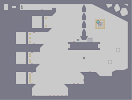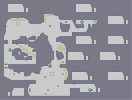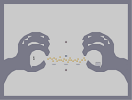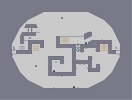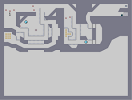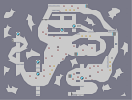63-0 Steel 63-1 Big thing Scary hand / Episode List 2 63-2 Tentakel 63-3 Bioway Chapter 1 Security

Pages: (0)

the lighter shades need to be lighter and the darker parts need to be darker. looks a bit too undefined, and 'cabbage-leafy'.

### Ist doch ganz einfach...

...ich hatte 580 Maps und hab 127 wieder veröffentlicht.
Deshalb hatte ich 707.

### Would you like to collab?

I want to better my object placement.

### seen this a million times

done the same way every time

happy valentines day though, 4
Looks exactly like the rose I got for my girlfriend yesterday. I'm pretty sure it looks more like a rose than it does a shell with a starfish or a cabbage so maybe you guys should just settle down. Great effort and if my oppinion mattered I say you did a really good job (: 4.5^ faved.

5/5

### nah

i doubt it really :3

i'll shut up now

### it

looks like a shell... with a starfish... :S
yes I do
two times
the first I lose because the flash player can´t load so much objekts
this looks very two dimensional now that i pay more attention
I like this robbage.
yeah, looks likes a really nice cabbage
did you do this yourself?

### Beautiful

It´s very nice

but red would be better I think because u cant see the rose very well